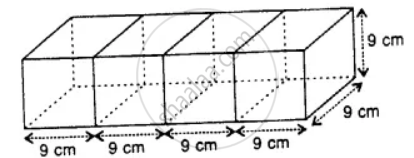# Four Cubes, Each of Edge 9 Cm, Are Joined as Shown Below :Write the Dimensions of the Resulting Cuboid Obtained. Also, Find the Total Surface Area and the Volume - Mathematics

Sum

Four cubes, each of edge 9 cm, are joined as shown below :Write the dimensions of the resulting cuboid obtained. Also, find the total surface area and the volume

#### Solution

Edge of each cube = 9 cm

(i) Length of the cuboid fonned by 4 cubes (l) = 9 x 4 = 36 cm
Breadth (b) = 9 cm and height (h) = 9 cm

(ii) Total surface area of the cuboid = 2(lb + bh + hl)
= 2 (36 x 9 + 9 x 9 + 9 x 36) cm2
= 2 (324 + 81 + 324) cm2
= 2 x 729 cm2
= 1458 cm2

(iii) Volume = l x b x h = 36 x 9 x 9 cm2 = 2916 cm3

Is there an error in this question or solution?
Chapter 21: Surface Area, Volume and Capacity - Exercise 21 (A) [Page 238]

#### APPEARS IN

Selina Concise Mathematics Class 8 ICSE
Chapter 21 Surface Area, Volume and Capacity
Exercise 21 (A) | Q 12 | Page 238
Share